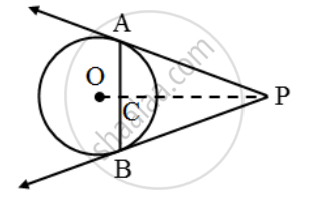# Prove that the tangents at the extremities of any chord make equal angles with the chord. - Mathematics

Sum

Prove that the tangents at the extremities of any chord make equal angles with the chord.

#### Solution

Let AB be a chord of a circle with centre O, and let AP and BP be the tangents at A and B respectively.Suppose the tangents meet at P. Join OP. Suppose OP meets AB at C. We have to prove that ∠PAC = ∠PBC In triangles PCA and PCB, we have

PA = PB

[∵ Tangents from an external point are equal]

∠APC = ∠BPC

[∵PA and PB are equally inclined to OP] and, PC = PC [Common]

So, by SAS – criterion of congruence, we have

∆PAC ≅ ∆PBC

⇒ ∠PAC = ∠PBC

Concept: Concept of Circle - Centre, Radius, Diameter, Arc, Sector, Chord, Segment, Semicircle, Circumference, Interior and Exterior, Concentric Circles
Is there an error in this question or solution?
Share